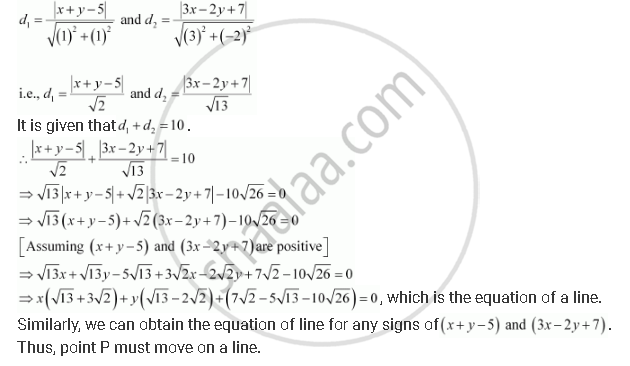CBSE (Arts) Class 11CBSE
Share

# If Sum of the Perpendicular Distances of a Variable Point P (X, Y) from the Lines X + Y – 5 = 0 and 3x – 2y + 7 = 0 is Always 10. Show that P Must Move on a Line. - CBSE (Arts) Class 11 - Mathematics

ConceptDistance of a Point from a Line

#### Question

If sum of the perpendicular distances of a variable point P (x, y) from the lines x + y – 5 = 0 and 3x – 2y+ 7 = 0 is always 10. Show that P must move on a line.

#### Solution

The equations of the given lines are

x + y – 5 = 0 … (1)

3x – 2y + 7 = 0 … (2)

The perpendicular distances of P (xy) from lines (1) and (2) are respectively given byIs there an error in this question or solution?

#### Video TutorialsVIEW ALL 

Solution If Sum of the Perpendicular Distances of a Variable Point P (X, Y) from the Lines X + Y – 5 = 0 and 3x – 2y + 7 = 0 is Always 10. Show that P Must Move on a Line. Concept: Distance of a Point from a Line.
S